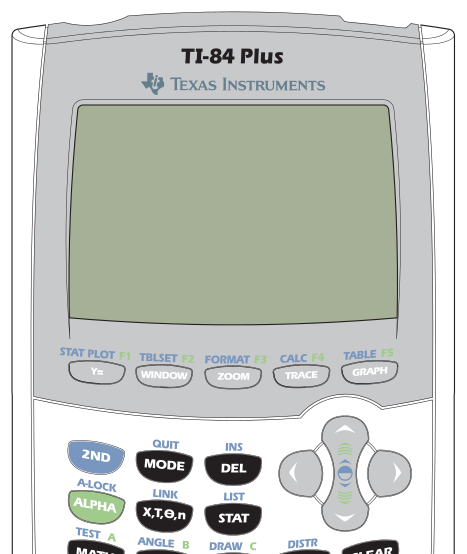# LAHS Math Department

The mathematics department supports and implements the philosophy of Laguna-Acoma Junior/Senior High School, and addresses the curriculum standards established by the New Mexico Public Education Department (NMPED).

The mathematics instruction is designed to develop strength in mathematics and to develop skills in both reasoning and problem solving, enabling students to approach questions in other academic areas as well as in life situations logically, rationally, and analytically. Instruction is sensitive to the unique needs of the students.

On this site you can find links to information about the mathematics department, the courses offered at Laguna-Acoma Junior/Senior High School, as well as where you can find help for your math courses.

## UNM-PNM Statewide Mathematics Competition

LAHS will be participating in this year's UNM-PNM Statewide Mathematics Competition! It is free, and just for fun. If your student is interested in participating, have them reach out to their LAHS math teacher!## Calculators

Technology provides dynamic opportunities for instruction in math and STEM classrooms. We can enhance the learning process and make concepts come alive through engaging and interactive media. Research has shown that students who have frequent access to handheld graphing calculators exhibit higher levels of mathematics achievement. The LAHS Mathematics Department utilizes TI-84 Plus graphing calculators in the classroom. We highly recommend that students purchase their own TI-84 Plus CE Graphing Calculator which is one of the latest products of the TI Education Technology to give them frequent access to the technology and help them increase their achievement.

## Common Core State Standards in Math

As a department, we are committed to making our instruction standards-based. According to New Mexico requirements, we use the Common Core State Standards. This document describes the Common Core recommendations for organizing high school courses.

## Course Descriptions

### Algebra 1

This is a one-year Algebra course intended for students who have been previously introduced to the basic arithmetic and geometric concepts and functions. This course emphasizes the learning of the four basic operations of real numbers, solving of one-step and multi-step equations, open sentences, functions and relations, variations, polynomials, algebraic fractions, special products, and factoring, graphing, slopes, inequalities, irrational numbers, and the quadratic equation.

Prerequisite(s): Math 8

### Geometry

This course is designed to emphasize the study of the properties and applications of common geometric figures in two and three dimensions. It includes the study of transformations and right triangle trigonometry. Inductive and deductive thinking skills are used in problem-solving situations, and applications to the real world are stressed. It also emphasizes writing proofs to solve (prove) properties of geometric figures. Students who complete Geometry should take Algebra II next.

Prerequisite(s): Algebra 1

### Algebra 2

Algebra II starts with a continuation of concepts studied in Algebra I. Topics include inequalities and absolute value, linear equations and systems, products and factors of polynomials, rational expressions, irrational numbers, quadratic equations and functions, analytical geometry, trigonometry and statistics/probability.

Prerequisite(s): Algebra 1 and Geometry

### Pre-Calculus

Pre-Calculus is a college and technical school preparatory course which covers essentially the same topics covered in trigonometry and an advanced college algebra course. These topics include: circular functions, trigonometric exponential and logarithmic functions, function analysis and applications.

Prerequisite(s): Algebra 2/Geometry passed with "C" or higher and teacher recommendation

Could be elective or required.

College credit if AP test is passed with 3+ points

### Trigonometry

This course will prepare students for eventual work in Pre-Calculus and Calculus. It includes review of linear and quadratic functions, trigonometric and circular functions (inverses and graphs), and relations among the parts of the triangle. It also includes the study of trigonometric identities and equations, solutions of right and oblique triangles, complex numbers, vectors, graphing on the polar coordinate system, matrix algebra, equations and graphs of conic sections, rotations and transformations, and parametric equations. Students completing this course can go into Pre-Calculus with a greater success rate.

Prerequisite(s): Grade of C or better in Geometry and Algebra 2.

### Math Intervention

Math Intervention is an extension of the regular grade level course that provides students who need additional focused instruction and support. This allows all students access to the regular curriculum with the support they need to be successful.

Goals of Math Intervention:

1. To increase student self-efficacy in mathematics (In other words: students begin to believe they can improve their math skills, they can actually do math when given more time and instruction, and they can be successful at learning mathematics.)

2. To support students in mastering grade level math objectives

3. To fill prerequisite grade skill gaps